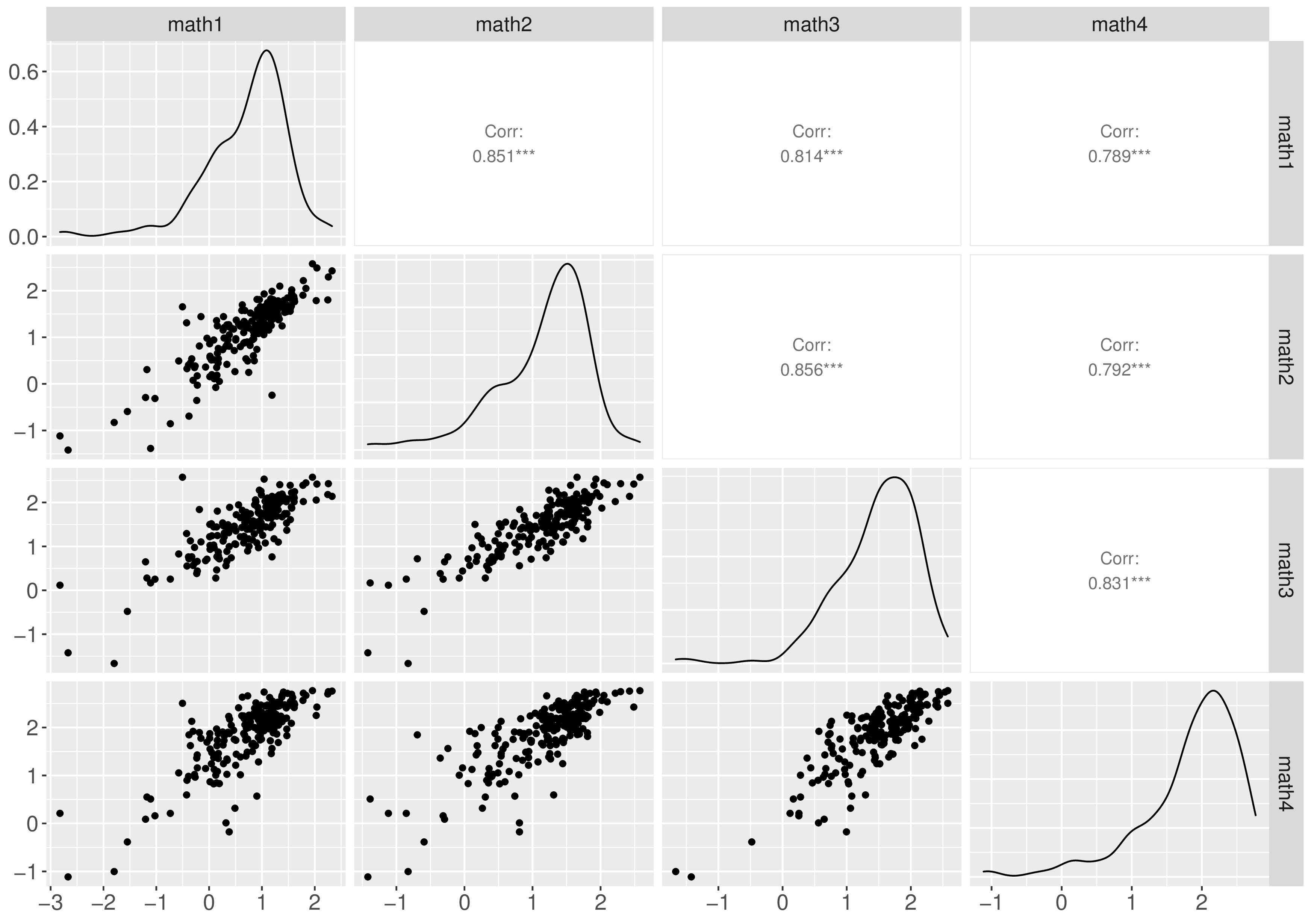# Robust Bayesian growth curve modeling: A tutorial using JAGS

## Authors

• Ruoxuan Li University of Notre Dame Author

## Keywords:

Robust Growth Curve Modeling, Bayesian Estimation, Structural Equation Modeling, JAGS

## Abstract

Latent growth curve models (LGCM) are widely used in longitudinal data analysis, and robust methods can be used to model error distributions for non-normal data. This tutorial introduces how to model
linear, non-linear, and quadratic growth curve models under the Bayesian framework and uses examples to illustrate how to model errors using t, exponential power, and skew-normal distributions. The code of JAGS models is provided and implemented by the R package runjags. Model diagnostics and comparisons are briefly discussed.